## Text Generation with LSTMs and GRUs

In this video we introduce text generation with LSTMs. A fun application with many practical uses.

Project

Build a text generator at https://github.com/lukas/ml-class/tree/master/projects/7-text-generator

Topics

• Limitations of RNNs
• How LSTMs work
• Gated Recurrent Units (GRUs)

## Text Generation

In this tutorial, we are going to learn to generate text using LSTMs – perhaps the most fun application of machine learning.

## One Hot Encoding

How do we convert text into the machine learning API? First we have to one hot encode our text. We covered this in the second tutorial, but as a recap, this means that we take each character and make a vector in which each element corresponds to a letter.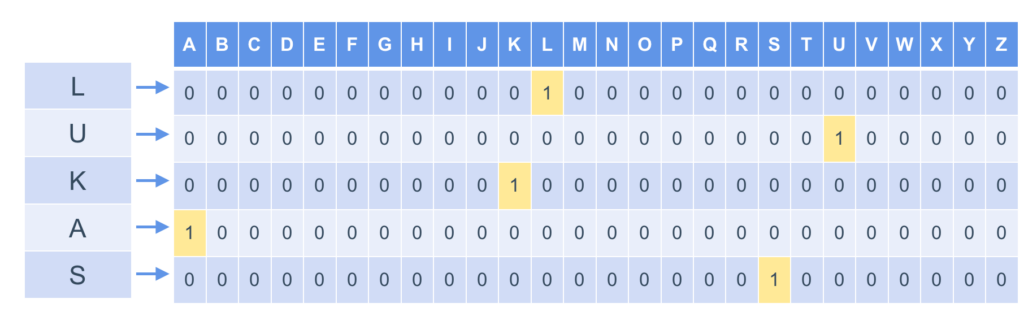One hot encoding characters

## Using Text Data

We feed each of these input vectors into our neural network, which outputs a vector. The output vector is also a one-hot encoded vector of the same length as our input vectors, so we can translate the output back into a character. We want our network to output the next letter at each step.## Code

Go into the directory text-gen and open up the file char-gen.py. Let’s walk through it:If we run the model, it seems to work fine. Why does this algorithm need improvement?

## The problem with RNNs

The problem with RNNs is that its really hard to encode long range dependencies, which is a result of how the network is constructed. Every new state is the previous state multiplied by a bunch of weights. This means that if the weight is even slightly less than one, at each step, the state is going to decrease. Over repeated multiplication at many steps, that value for state would go down to 0. Conversely, if the weight was even slightly more than one, very quickly that state value would explode. This makes it really hard to ‘remember’ state over a longer range.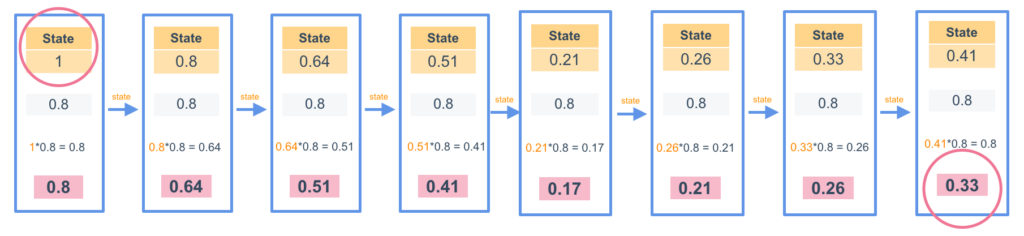The problem with RNNs: state decays very quickly with repeated multiplication

For instance, if we have the input of a left parenthesis, we know that there should be a right parenthesis in max 15-20 characters. However, it is really hard for the RNN to remember the state of the open parenthesis by the time it gets to 15-20 characters later, as the state would be multiplied by a number less than 1 over and over again.

## Long Short Term Memory Models (LSTMs)

Long Short Term Memory Models (LSTMs) were invented to try and solve this problem. Let’s take a look into how they work.

Just like simple RNN’s, LSMTs take in a vector and output a vector. Unlike RNNs however, the state passed to the next iteration is different to the output at each step. LSTMs do a bunch of precalaculations before they decide what to output and what to pass along as state. They perform four independent calculations using perceptrons at each step, and use the result of those calculations to decide the new state and the output. The four calculations are Forgetting (Ft), Update Activation (It), Candidate (C~t) and Output Activation (Ot). Let’s go through them.Inside an LSTM: 4 calculated vectors decide what to output and what to pass as state

## Forgetting (Ft)

The first calculation LSTMs do is what to forget. In order to calculate Ft, the input is concatenated with the previous state in the LSTM, and fed into a dense layer. The output from the dense layer is the same size as the previous state (in this case 1×3). Next, a sigmoid activation function is run on the output to transform all values into a number between 0 and 1. Here, 1 means that the LSTM should keep the previous state value, and 0 means that the LSTM should forget the previous state value. We will see how this works in a minute.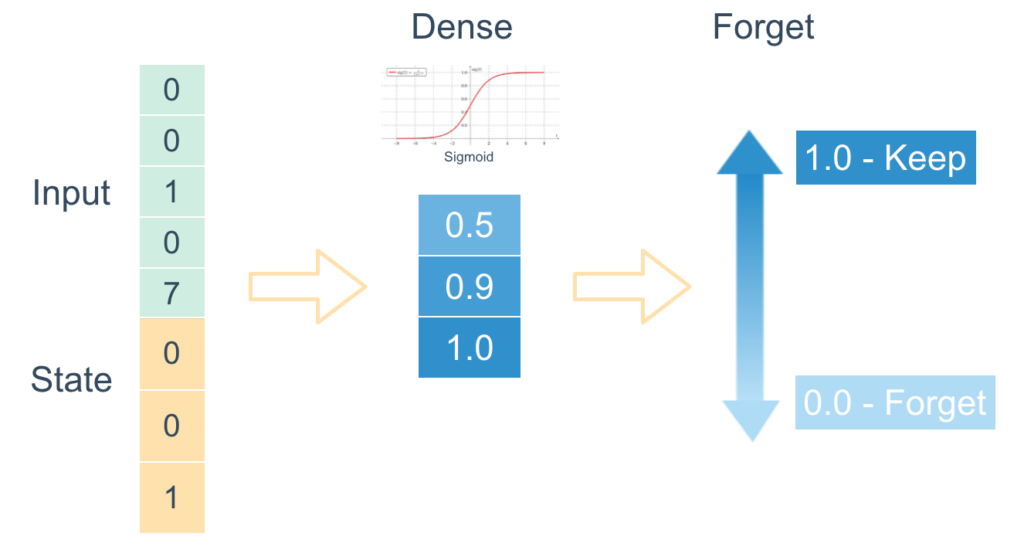## Update Activation(It)

The second calculate the LSTM does is update. This works the same as the forget calculation, but the perceptron has different weights so will output a different vector, which is also the same size as the state vector (1×3). Here we interpret the values as 1 being values that the LSTM should update, and 0 being values the LSTM should not update.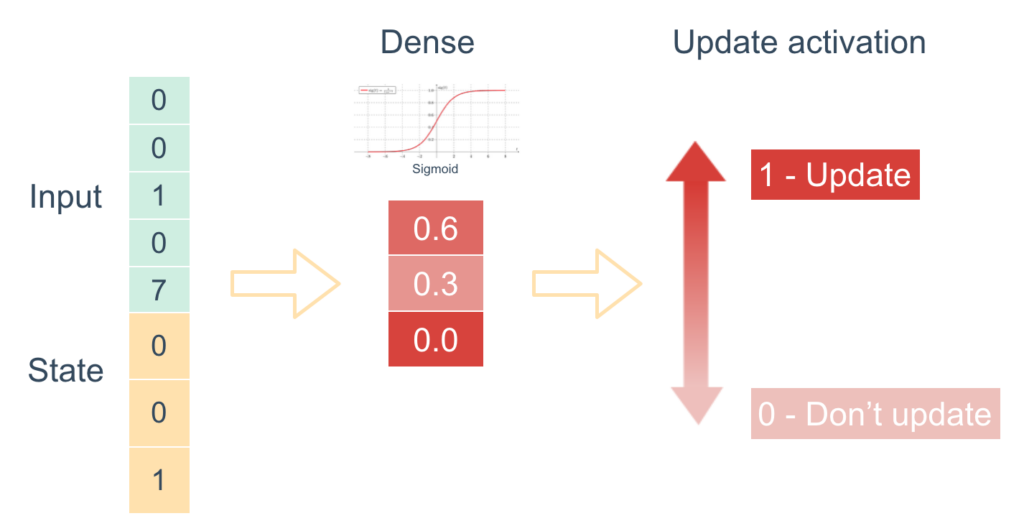## Candidate (C~t)

The third calculation the LSTM does is to decide what updated values the LSTM might want to use, called Candidate. Again, this is the same perceptron calculation as we did previously, but this time, the perceptron uses a hyperbolic tangent function, so the output values are between -1 and 1. This is interpreted as 1 being a large positive change to the state, 0 being no change, and -1 being a large negative change.## Output Activation (Ot)

The final calculation the LSTM does is to decide what it should be outputting. Again, this takes in the input concatenated with the previous state and runs a perceptron with a sigmoid activation function. Here 1 means that it should output that value, and 0 means it should not output the value.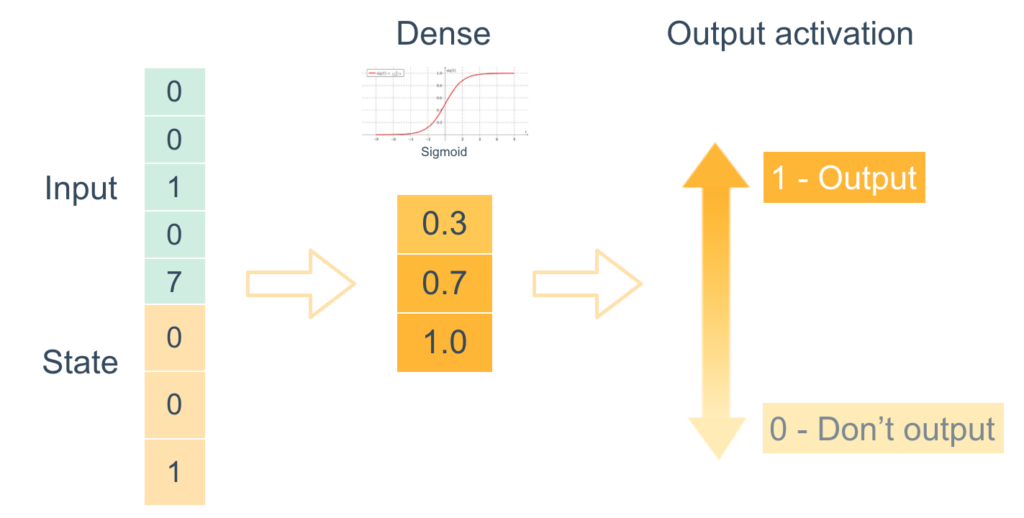## Putting it all together

We combine all of these values in the following equation, where Output (Ht) is what is outputted and the next state (Ct) is what is passed to the next iteration.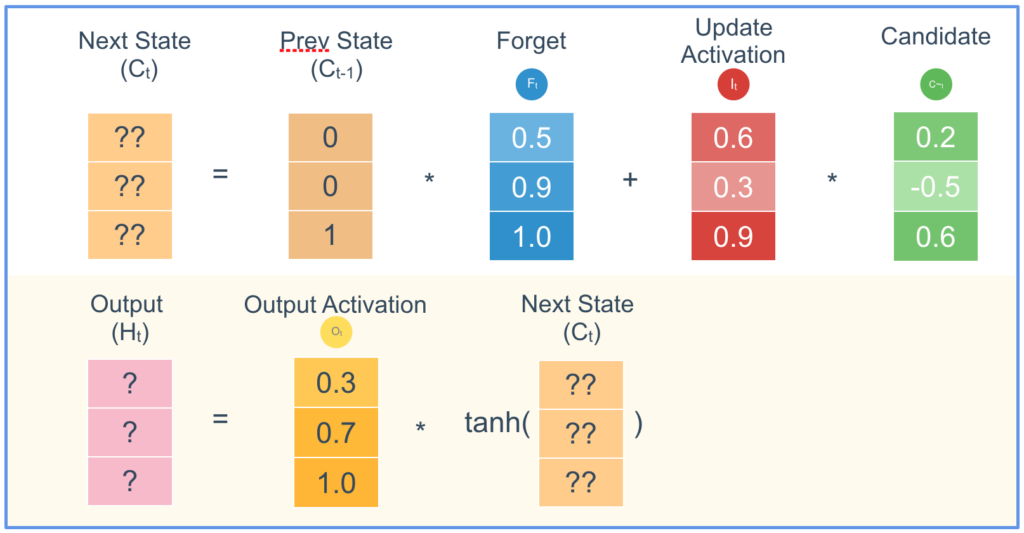All of the multiplications here are element-wise multiplication. Let’s look at the first equation. We can think of next state as equal to (remembered values of the previous state) + (updated values).

Where the forget vector is set to 0, the values from the previous state are effectively removed (forgotten) and where the forget vector is set to 1, the values from the previous state are preserved (remembered). Next, the LSTM thinks about how it wants to update the state values. When the update activation is set to 0, it doesn’t update the state value, and when the update activation is set to 1, it does a complete update with the new candidate value. This makes it really easy for an LSTM to encode long-term memory – as long as the forget value is set to 1 and the update activation is set to 0,  the next state will have the same value as in the previous state.

Now take a look at the second equation. This determines what the cell will output. The output value will be equal to the output activation multiplied by the hyperbolic tangent of the next state that we just calculated. This means that the output does not have to be the same as the next state.

These calculations are important for 2 main reasons: they allow the LSTM to encode long-term memory, and are an efficient structure to perform backpropagation. Calculating all the different parameters for each perceptron is an expensive process, but these computations are optimized to make these processes as efficient as possible. However, since there are so many parameters, LSTMs can be prone to overfitting.

## LSTM Code

It’s actually really easy to add LSTMs to your code. Open up char-gen.py and on line 62, replace the RNN layer with an LSTM layer:

// line 62

Ok that was a lot. If you’re thinking that there must be a more simple way to do this calculation, you’re right – that’s exactly what Gated Recurrent Units do.

## Gated Recurrent Units (GRUs)

Gated Recurrent Units were invented in 2014, and are a simplified version of LSTMs that can train a little faster with often no loss in performance. There are 2 big differences between a GRU and an LSTM.

Firstly, the output values are the same values as the state that it passes into the next step.Secondly, there are only three computations that we are doing, not four. If we look at LSTMs, we have a different vector for what to forget and what to update. This may seem a little redundant – if we forget the previous value, we want to update it, and if we don’t forget the previous value we don’t want to update it. GRUs merge these two vectors into a single vector – the forget value is just (1 – the update activation).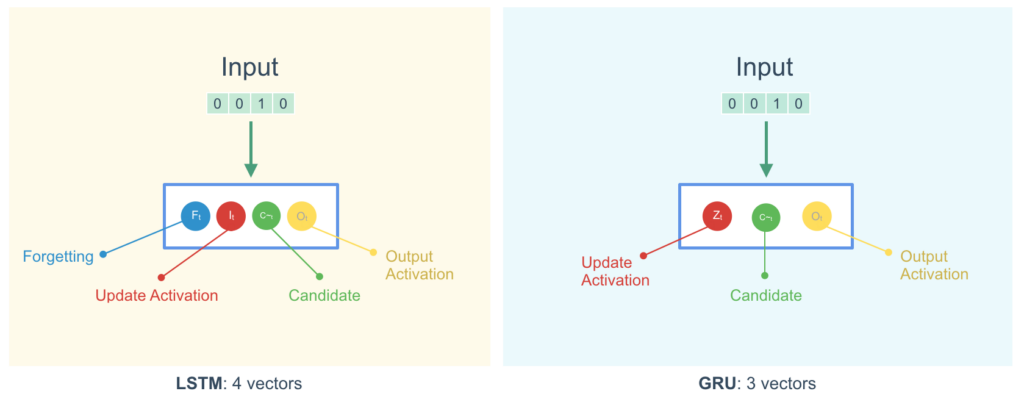We can go back into the code and easily change our LSTM into a GRU. We just change the LSTM layer into a GRU layer:

// line 62